Next: Nonlinearly active modes and Up: Results Previous: Frequency properties.

## Amplitude and phase evolution.

 [width=10cm]k2500_amp.eps
 [width=10cm]k2500_phase.eps
 [width=10cm]k2500_ampDetail.eps

Figures 7 and 8 show time evolution of the amplitude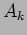and the (interaction representation) phase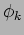respectively for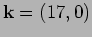. The phase evolution seen in figure 8 consists of the time intervals when it oscillates quasi-periodically (with amplitude less than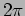) which are inter-leaved by sudden phase runs'', - fast monotonic phase changes by values which can significantly exceed. By juxtaposing figures 7 and 8, one can see that when the phase runs happen then the amplitude is close to zero. We zoom in at the amplitude graph in a time interval characterized by low amplitudes (and therefore phase runs) in figure 9. One can see large-amplitude quasi-periodic oscillations on, - it changes in value several-fold over a time comparable to the linear wave period. This indicates that such phase run intervals mark the places where the WT assumption of weak nonlinearity breaks down.

This behavior, together with the two-peak character of the time-Fourier spectrum, suggest that at each wavenumber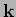there are two modes: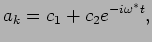where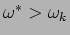and the complex amplitudes are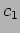and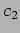varying in time slower than the linear oscillations. Most of the time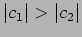and, therefore, the phaseoscillates periodically about the some mean value (equal to the phase of). However, sometimesbecomes greater thanand then the path of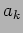will encircle zero in the complex plane, so thatstarts gainingfor each rotation. These are the phase runs, and in order to trigger these runs the complex-plane path ofmust encircle zero, which explains the observed small values of this quantity during the phase runs. This effect is related to the general phenomenon of phase singularities of complex fields at points of zero amplitude (e.g. ). However, our phase runs continue all the time untilbecomes greater thanand during this time interval (excluding its ends) the amplitude is non-zero and the phase is perfectly regular.

Note that such observed behavior of the phase is very dependent on the wave variables: it occurs in natural variables such as the surface height and velocity but is absent for the waveaction variable after the canonical transformation leading to the Zakharov equation. Thus, the phase runs can say nothing about the dynamics or statistics of the waveaction amplitude used in WT, but they mark short time intervals when nonlinearity fails to be weak for a particular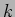-mode, and this will be used as a diagnostic tool in our simulations.

 [width=10cm]phase_runs.eps

In figure 11 we show comparison of the mean rate of the phase change during the upward phase runs and the second peak's frequency at different wavenumbers. A significant coincidence of these two curves supports the proposed above two-mode explanation.

 [width=10cm]HighFrequencyPeak.eps

A word of caution is due about the simple two-mode explanation of the phase runs. Indeed, according to this picture the phase should always run to higher values whereas in figure 8 we see both upward and downward runs. A possible explanation of this is that phase runs may be triggered not only by sharp peaks but also by broadband distributions with frequencies less than the linear one. Such broadband distributions could be made of modes which are strong (and therefore can produce phase runs) but whose duration in time is short and sporadic and at different frequencies (hence a broad spectrum). This picture is supported by the fact that the amount of total wave energy in the frequency range below the linear frequency is similar (and for low wavenumbers even greater) than the amount of energy in the range above the linear frequencies, see figure 13. Evidence of relation of the sub-linear modes and the downward phase runs is shown in figure 12 where the mean rate of the phase change during the downward phase runs and the frequency of the highest sub-linear peak. Again, we can see agreement of these two curves although with a greater level of fluctuations due to the fact the sub-linear modes are very spread over different frequencies.

 [width=10cm]LowFrequencyPeak.eps

 [width=10cm]power.epsNext: Nonlinearly active modes and Up: Results Previous: Frequency properties.
Dr Yuri V Lvov 2007-01-16